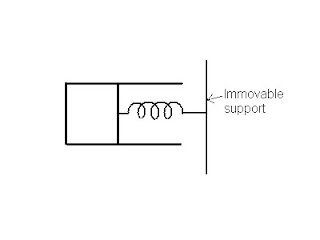## Pages

`“Life is like riding a bicycle.  To keep your balance you must keep moving.”–Albert Einstein`

## Thursday, October 28, 2010

### AP Physics B – Answer to Free Response Practice Question on Thermodynamics

“Our greatest weakness lies in giving up. The most certain way to succeed is always to try just one more time”

– Thomas A. Edison

A free response practice question on thermodynamics was posted on 24th October 2010 for AP Physics B aspirants. As promised, I give below a model answer (along with the question) for your benefit.The adjoining figure shows a fixed cylindrical vessel of inner diameter 10 cm fitted with a smooth, light piston connected to a light spring of spring constant 4000 Nm–1. The other end of the spring is attached to an immovable support. The cylinder contains an ideal gas at 27º C. The spring is initially in its released condition and the initial volume of the gas in the cylinder is 0.8×10–3 m3. When heat is supplied to the gas, it expands and pushes the piston through 10 cm. The atmospheric pressure is 105 pascal. Now answer the following questions:

(a) What are the initial pressure and the initial absolute (Kelvin) temperature of the gas?

(b) Calculate the potential energy acquired by the spring because of the expansion of the gas.

(c) Calculate the final pressure of the gas in the cylinder.

(d) Calculate the final temperature of the gas in the cylinder.

(a) The initial pressure P1 of the gas is the same as the atmospheric pressure 105 Nm–1. The initial temperature T1 of the gas is (27 + 273) K = 300 K.

(b) The potential energy of the spring is U = ½ kx2 where k is the spring constant and x is the compression of the spring.

Therefore, U = ½ ×4000×(0.1)2 = 20 J.

(c) The final pressure of the gas is the sum of the atmospheric pressure and the pressure due to the elastic force developed in the spring.

Elastic force = kx.

Since this force acts over the area A of the piston, pressure due to the elastic force is kx/A = kx/πR2 where R is the radius of the piston.

Therefore, final pressure P2 of the gas = 105 + (4000×0.1)/(π×0.052) = 105 + 0.51×105 = 1.51×105 pascal.

(d) The final temperature T2 of the gas is given by

P1V1/T1 = P2V2/T2

Therefore, T2 = (P2V2 T1)/ (P1V1)

The final volume V2 of gas = Initial volume + πR2x = 0.8×10–3 + (π×0.052×0.1) = 0.8×10–3 + 0.79×10–3 = 1.59×10–3 m3

Therefore, final temperature T2 = (1.51×105×1.59×10–3 ×300)/(105×0.8×10–3) = 900 K.